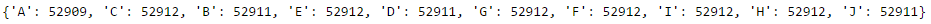# TensorFlow Udacity 1_notmnist - Part 3### Summary of 1_notmnist

Basically 1_notmnist is to learn how to display data in Jupyter Notebook. Besides, it also let us know on sklearn - a python machine library - so that we can then compare with TensorFlow. This is the exact ipynb file at Tensorflow Github Repo.

### Notice

This is as a form of sharing and discuss on better way to solve 1_notmnist problem. Do not copy and paste directly as it does not help on improving yourself + the answer is not optimized.

The entire series of TensorFlow Udacity can be found at here

### Solving Problem 3

len_dict = {}
for folder in folder_names:
t = pickle.load(open(dir_name + "/" + folder + ".pickle", "r"))
len_dict[folder] = len(t)
print(len_dict)### Comment

Since the image for each class is roughly around 52911, therefore we can say that the data is balanced. Though more appropiate way is to calculate the standard deviation :)

Written on April 2, 2016